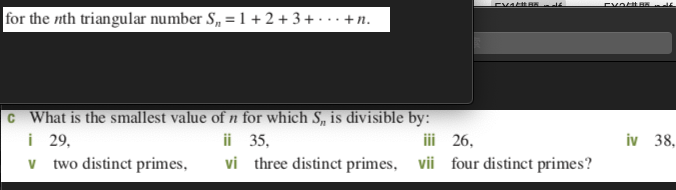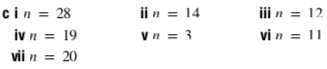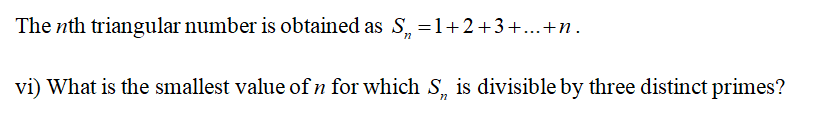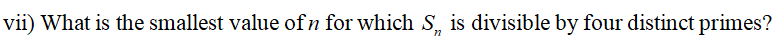# for the nth triangular number S, =1+2+3+ • .. +n.What is the smallest value of n for which S, is divisible by:i 29,v two distinct primes,iv 38.ii 35,iii 26,vi three distinct primes,viifour distinct primes? cin = 28iv n = 19ii n = 14iii n = 12vi n = 11vii n = 20%3D

Question
95 views

Answers attached. Please solve c) vi and vii with full explained working. Thank you.help_outlineImage Transcriptionclosefor the nth triangular number S, =1+2+3+ • .. +n. What is the smallest value of n for which S, is divisible by: i 29, v two distinct primes, iv 38. ii 35, iii 26, vi three distinct primes, vii four distinct primes? fullscreenhelp_outlineImage Transcriptionclosecin = 28 iv n = 19 ii n = 14 iii n = 12 vi n = 11 vii n = 20 %3D fullscreen
check_circle

Step 1Step 2...

### Want to see the full answer?

See Solution

#### Want to see this answer and more?

Solutions are written by subject experts who are available 24/7. Questions are typically answered within 1 hour.*

See Solution
*Response times may vary by subject and question.
Tagged in

### Math